BASH Programming

# Bash lowercase and uppercase strings

String data are used for different purposes in any bash commands or programming script. Sometimes we need to change the case of the string to get the desired output. The string can be converted to uppercase or lowercase. The string data is converted by using ‘tr’ command in the old version of bash. In this case, the keyword ‘: upper’ is used for uppercase and the keyword ‘: lower’ is used for lowercase. You can visit the following tutorial link to know more about the ‘tr’ command for converting the case of the string.

You can convert the case of the string more easily by using the new feature of Bash 4. ‘^’ symbol is used to convert the first character of any string to uppercase and ‘^^’ symbol is used to convert the whole string to the uppercase. ‘,’ symbol is used to convert the first character of the string to lowercase and ‘,,’  symbol is used to convert the whole string to the lowercase.

## Converting the case of the String

### Example#1:

Run the following commands to assign a string input to the variable, \$name, and the next commands are used to print the original value, print value by converting the first letter to uppercase and print value by converting all letters of the string to uppercase.

\$ name='fahmida'
\$ echo \$name
\$ echo \${name^}
\$ echo \${name^^}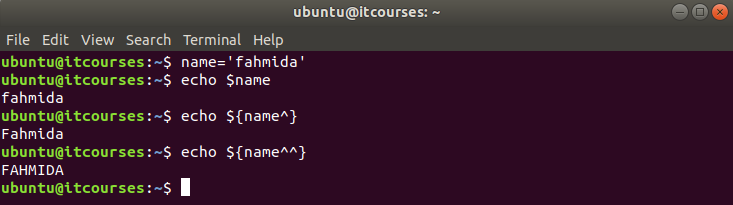### Example#2:

The following example shows how you can convert the first character of any string to uppercase by matching with a particular character.  Here, the first character is compared with ‘l’ and ‘h’ by the last two commands.

\$ site='linuxhint'
\$ echo \$site
\$ echo \${site^l}
\$ echo \${site^h}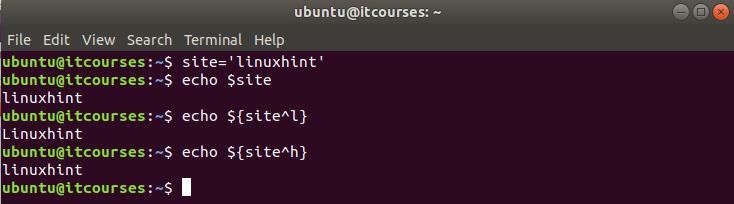### Example#3:

In the following example, \$language variable is used to store a text value and the third command is used to covert the first character of each word of the string to uppercase where the first character is ‘p’. The last command is used to match the first character of each word of the text with ‘p’ and ‘j’ and convert them to uppercase.

\$ language='python perl java php c#'
\$ echo \$language
\$ echo \${language^^p)}
\$ echo \${language^^[p,j]}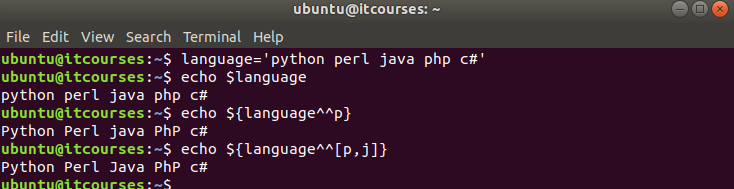### Example#4:

Create a base file named case1.sh with the following code. In this example, the user input is taken in the variable, \$ans and the value of this variable is printed with other string by converting the first character to uppercase.

#!/bin/bash
read -p "Do you like music? " ans

Run the script.

\$ bash case1.sh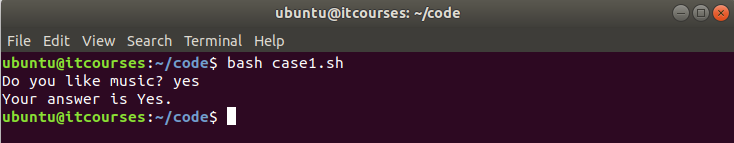### Example#5:

Create a bash file named case2.sh with the following code.  The string value taken from the user is converted to uppercase and stored to the variable \$answer. If the value of this variable matches to ‘ADD’ then the value of \$a, and \$b will be added and printed. If the value of this variable matched to ‘SUBTRACT’ then the subtraction result of \$a, and \$b will be printed. The script will print ‘Invalid answer’ if the value provided by the user doesn’t match with ‘ADD’ or ‘SUBTRACT’.

#!/bin/bash
a=15
b=20
elif [ \$answer == 'SUBTRACT' ]; then
echo "The result of subtraction=\$((a-b))"
else
fi

Run the script.

\$ bash case2.sh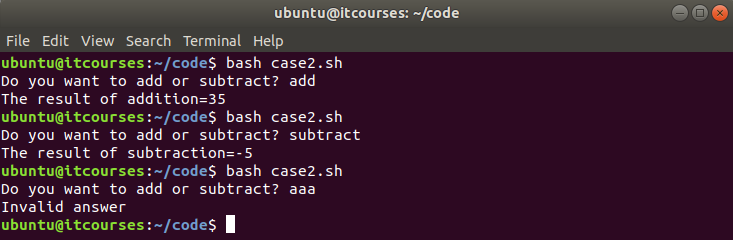### Example#6:

Create a bash file named case3.sh with the following script. In this example, a text value is taken from the user and stored into the variable \$data. Next, the comma-separated character list is taken as input for the case conversion and stored into the variable \$list. The variable is used to match the characters of the list with the value of \$data. The script will print the output after converting the characters to uppercase where matches.

#!/bin/bash
read -p "Enter some text data: " data
read -p "Mention the letters with the comma that will convert to uppercase?: " list
echo -n "The highlighted text is :  "
echo \${data^^[\$list]}

Run the script.

\$ bash case3.sh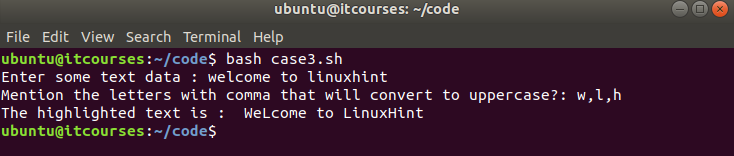### Example#7:

Create a bash file named case4.sh with the following code. Here, ,, operator is used to convert the values taken from the users and compare with the variable \$username and \$password. If both values match then the script will print “Valid user” otherwise it will print “Invalid user”.

#!/bin/bash
user=\${u,,}
pass=\${p,,}
if [ \$username == \$user ] && [ \$password == \$pass ]; then
echo "Valid User"
else
echo "Invalid User"
fi

Run the script.

\$ bash case4.sh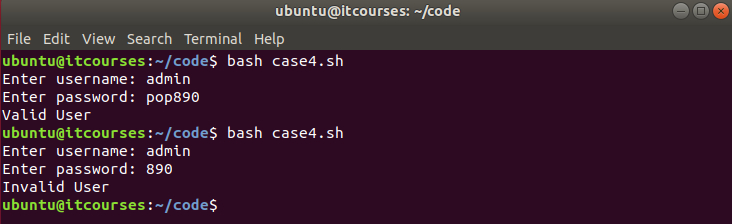#### Conclusion:

Hope, this tutorial will help you to learn the case conversion tasks in easier way by using the new feature of bash. For more information watch the video!